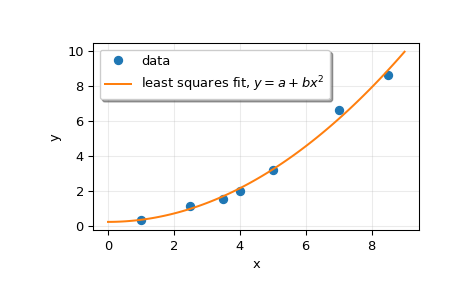# scipy.linalg.lstsq¶

scipy.linalg.lstsq(a, b, cond=None, overwrite_a=False, overwrite_b=False, check_finite=True, lapack_driver=None)[source]

Compute least-squares solution to equation Ax = b.

Compute a vector x such that the 2-norm |b - A x| is minimized.

Parameters: a : (M, N) array_like Left hand side matrix (2-D array). b : (M,) or (M, K) array_like Right hand side matrix or vector (1-D or 2-D array). cond : float, optional Cutoff for ‘small’ singular values; used to determine effective rank of a. Singular values smaller than rcond * largest_singular_value are considered zero. overwrite_a : bool, optional Discard data in a (may enhance performance). Default is False. overwrite_b : bool, optional Discard data in b (may enhance performance). Default is False. check_finite : bool, optional Whether to check that the input matrices contain only finite numbers. Disabling may give a performance gain, but may result in problems (crashes, non-termination) if the inputs do contain infinities or NaNs. lapack_driver : str, optional Which LAPACK driver is used to solve the least-squares problem. Options are 'gelsd', 'gelsy', 'gelss'. Default ('gelsd') is a good choice. However, 'gelsy' can be slightly faster on many problems. 'gelss' was used historically. It is generally slow but uses less memory. New in version 0.17.0. x : (N,) or (N, K) ndarray Least-squares solution. Return shape matches shape of b. residues : (0,) or () or (K,) ndarray Sums of residues, squared 2-norm for each column in b - a x. If rank of matrix a is < N or N > M, or 'gelsy' is used, this is a lenght zero array. If b was 1-D, this is a () shape array (numpy scalar), otherwise the shape is (K,). rank : int Effective rank of matrix a. s : (min(M,N),) ndarray or None Singular values of a. The condition number of a is abs(s / s[-1]). None is returned when 'gelsy' is used. LinAlgError If computation does not converge. ValueError When parameters are wrong.

optimize.nnls
linear least squares with non-negativity constraint

Examples

>>> from scipy.linalg import lstsq
>>> import matplotlib.pyplot as plt


Suppose we have the following data:

>>> x = np.array([1, 2.5, 3.5, 4, 5, 7, 8.5])
>>> y = np.array([0.3, 1.1, 1.5, 2.0, 3.2, 6.6, 8.6])


We want to fit a quadratic polynomial of the form y = a + b*x**2 to this data. We first form the “design matrix” M, with a constant column of 1s and a column containing x**2:

>>> M = x[:, np.newaxis]**[0, 2]
>>> M
array([[  1.  ,   1.  ],
[  1.  ,   6.25],
[  1.  ,  12.25],
[  1.  ,  16.  ],
[  1.  ,  25.  ],
[  1.  ,  49.  ],
[  1.  ,  72.25]])


We want to find the least-squares solution to M.dot(p) = y, where p is a vector with length 2 that holds the parameters a and b.

>>> p, res, rnk, s = lstsq(M, y)
>>> p
array([ 0.20925829,  0.12013861])


Plot the data and the fitted curve.

>>> plt.plot(x, y, 'o', label='data')
>>> xx = np.linspace(0, 9, 101)
>>> yy = p + p*xx**2
>>> plt.plot(xx, yy, label='least squares fit, $y = a + bx^2$')
>>> plt.xlabel('x')
>>> plt.ylabel('y')